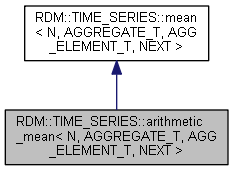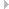RDM::TIME_SERIES::arithmetic_mean< N, AGGREGATE_T, AGG_ELEMENT_T, NEXT > Class Template Reference

Template Class for doing an arithmetic mean. More...

`#include "rdm_time_series_mean.h"`

Inheritance diagram for RDM::TIME_SERIES::arithmetic_mean< N, AGGREGATE_T, AGG_ELEMENT_T, NEXT >:Collaboration diagram for RDM::TIME_SERIES::arithmetic_mean< N, AGGREGATE_T, AGG_ELEMENT_T, NEXT >:Public Member Functions inherited from RDM::TIME_SERIES::mean< N, AGGREGATE_T, AGG_ELEMENT_T, NEXT >
RDM_RETCODE init (RDM_DB db)
Initialize this object. More...
void reset (void)
Reset this object. More...
RDM_RETCODE flush_value (uint32_t threshold=1, RDM::DB::TRANSACTIONAL_T transactional=RDM::DB::NOT_TRANSACTIONAL)
Flush this object. More...
RDM_RETCODE flush_range (uint32_t threshold=1, RDM::DB::TRANSACTIONAL_T transactional=RDM::DB::NOT_TRANSACTIONAL)
Flush this object. More...
template<class SOURCE_VALUE_T >
RDM_RETCODE put_value (SOURCE_VALUE_T *source_value, RDM::DB::TRANSACTIONAL_T transactional=RDM::DB::NOT_TRANSACTIONAL)
Template method for receiving a data values. More...
template<class SOURCE_AGGREGATE_T >
RDM_RETCODE put_range (SOURCE_AGGREGATE_T *source_range, RDM::DB::TRANSACTIONAL_T transactional=RDM::DB::NOT_TRANSACTIONAL)
Template method for receiving ranges. More...

## Detailed Description

### template<uint32_t N, class AGGREGATE_T, class AGG_ELEMENT_T, class NEXT> class RDM::TIME_SERIES::arithmetic_mean< N, AGGREGATE_T, AGG_ELEMENT_T, NEXT >

Template Class for doing an arithmetic mean.

Template class for taking the arithmetic means of data values or ranges. What is received will be collected and used for taking arithmetic means. The result of those arithmetic means will be forwarded to the next class in the chain.

Upon destruction of this class, there might be incomplete aggregations that have not yet been passed to the next class in the chain. flush() can be called to force those incomplete aggregations to be sent to the next class. This approach may not always be desirable. Another approach is to restore the state of this class the next time this class is instantiated. See restore_aggregate for details.

Template Parameters
 N The number of receved RANGE calculations for each arithmetic mean. AGGREGATE_T The aggregate range or stats class for which objects will be passed to the next class. AGG_ELEMENT_T The type for the elements that must be aggregated to compute the mean. NEXT The class in the chain to receive arithmetic means.
Examples
time_series_fft.cpp.

The documentation for this class was generated from the following file: